## Chapter 7Electroacoustic Measurements

### 7.1 Tools of the Trade

I could describe a piece of paper using a list of its measurements. If I said, “one sheet of white paper, 8.5 inches by 11 inches” you’d have a pretty clear idea of what I was talking about. The same can be done for audio gear – we can use various measurements to describe the characteristics of a given piece of equipment. Although it would be impossible to perform a set of measurements that describe the characteristics exactly, we can get a good idea of what’s going on.

Before we look at the various electroacoustic measurements you can perform on a piece of audio equipment, we’ll look at the various tools that we use.

#### 7.1.1 Digital Multimeter

A digital multimeter (also known as a DMM for short) is a handy device that replaces a number of different analog tools such as a voltmeter and a galvanometer. The particular tools incorporated into one DMM depends on the manufacturer and the price range.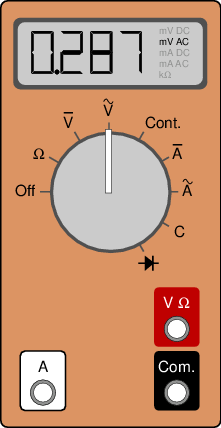Figure 7.1: A cheap digital multimeter. This thing is about the size of a credit card, not much thicker and cost around \$20Cdn.

At the very least, a DMM will have meters for measuring voltage and current – both AC and DC. These are typically chosen in advance, so you set the switch on the meter to “AC Voltage” to do that particular measurement, for example. In addition, the DMM will invariably include a meter for measuring the resistance of a device (such as a resistor, for example...) Usually, the last meter you’ll be guaranteed is a great little tool called a continuity tester. This is essentially a simplified version of a resistance meter where the only output of the meter is a “beep” when there is a low-resistance connection between the two leads.

Some fancier (and therefore more expensive) units will also include a diode tester and a meter that can measure capacitance.

There are a couple of things to be concerned about when using one of these devices.

First, we’ll just look a the basic operation. Let’s say that you wanted to measure the DC voltage between two points on your circuit. You set the DMM to a DC Voltage measurement and touch the two leads on the two points. Be careful not to touch more than one point on the circuit with each lead of the DMM. This could do some damage to your circuit... Note that one of the leads is labelled “ground” which does not necessarily mean that it’s actually connected to ground in your circuit. All it really means is that, for the DMM, that particular lead is the reference. The voltage displayed is the voltage of the other lead relative to the “ground” lead.

When you’re measuring voltage, the two leads of the DMM theoretically have an infinite impedance between them. This is necessary because, if they didn’t, you’d change the voltage that you were measuring by measuring it. For example, let’s pretend that you have two identical resistors in series across a voltage source. If your DMM had a small impedance across the two leads, and you used it to measure the voltage across one of the resistors, then the small impedance of the DMM would be in parallel with the resistor being measured. Consequently, the voltage division will change and you won’t get an accurate measurement. Since the impedance between the leads is Ω (or at least, pretty darned close...), when it’s in parallel with a resistor, it’s as if it’s not there at all, and it consequently has no effect on the circuit being measured.

If you’re measuring current, then you have to use the DMM a little differently. Whereas a voltage measurement is performed by putting the DMM in parallel with the resistor being measured, a current measurement is performed by putting the DMM in series with the circuit. This ensures that the current goes through the DMM on its way through the circuit. Also, as a result, it’s good to remember that the impedance across the leads for a current measurement is 0Ω – otherwise, it would change the current in the circuit and you’d get an inaccurate measurement.

Now, there’s an important thing to remember here – when the DMM is in voltage measuring mode, the impedance is infinity. When it’s in current measuring mode, it’s 0Ω. Therefore, if you are measuring current, and you accidently forget to set the meter back to voltage measuring mode to measure the voltage across a power supply or a battery, you’ll probably melt something. Remember that power supplies and batteries don’t like being shorted out with a 0Ω impedance – it means that you’re asking for infinite current...

Another thing: a DMM measures resistance (and continuity) by putting a small voltage difference across its leads and measuring the resulting current through it. The smaller the current, the bigger the resistance. This also means, however, that your DMM in this case, is its own little power supply – so you don’t want to go poking at a circuit to measure resistors without lifting the resistor out – one leg of the resistor will do.

One last thing to remember: the AC measurements in a typical DMM are supposed to be RMS measurements. In fact, they’re pseudo-RMS, so you can’t usually trust them. Remember from way back that the RMS of a sine wave is about 0.707 of its peak value? Well, a DMM assumes that this is always the case – so it measures the peak value, multiplies by 0.707 and displays that number. This is fine if all you ever do is measure sine waves (like most electricians do...) but we audio types deal with waveforms other than sinusoids, so we need to use something a little more accurate. Just remember, if you’re using a DMM and it’s not a sine wave, then you’re looking at the wrong answer.

A small thing that you can usually ignore: a DMM is only accurate (such as it is...) within a limited frequency range. Don’t expect a \$10 DMM to be able to give you a reliable reading on a 30 kHz sine tone.

#### 7.1.2 True RMS Meter

A true RMS meter is just a fancy DMM that actually does a real RMS voltage measurement instead of some quick and dirty short cut to the wrong answer. Unfortunately, because there’s not much demand for these things, they’re a LOT more expensive than a run-of-the-mill DMM. Bet on spending tens to hundreds of times the cost of a DMM to get a true RMS measurement. They look exactly the same as a normal DMM, but they have the words “True RMS” written on it somewhere – that and the price tag will be higher (on the order of about \$300 Cdn).

Note that a true RMS meter usually suffers from the same limited frequency range problem as a cheap DMM. The good thing is that a true RMS meter will have a much wider range, and the manual will probably tell you what that range is, so you know when to stop trusting it.

You may also see a DMM that has a switchable low-pass filter on it. This may seem a little silly at first, but it’s actually really useful for doing noise measurements. For example, if you want to measure the noise output of a microphone preamp, you’ll have to do a true RMS measurement, but you’re not really worried about noise above, say, 100 kHz (then again, maybe you are...). If you just did the measurement without a low pass filter, you’d get a much bigger value than you’ll like, and one that isn’t representative of the audible noise level. So, in this case, the low pass filter is your friend. If your true RMS meter doesn’t have one, you might want to build one out of a simple RC circuit. Just remember, though, that if you’re stating a noise measurement that you did, include the LPF magnitude characteristic with your values.

#### 7.1.3 Oscilloscope

Digital multimeters and true RMS meters are great for doing a quick measurement of a signal and getting a single number to describe it – the problem is that you really don’t know all that much about the signal’s shape. For example, if I handed you two wires and asked you to give me a measurement of their voltage difference with a true RMS meter, you’d tell me one, maybe two numbers (the AC and DC components can be measured separately, remember...). But you don’t know if it’s a sine wave or a square wave or a Britney Spears tune.

In order to see the shape of the voltage waveform, we use an oscilloscope. This is a device which can be seen in the background of every B science fiction film ever made. All evil scientists and aliens seem to have oscilloscopes in their labs measuring sine waves all the time.

An oscilloscope displays a graph showing the voltage being measured as it changes in time. It shows this by sweeping a bright little dot across the screen from left to right and moving it up when the voltage is positive and down when it’s negative.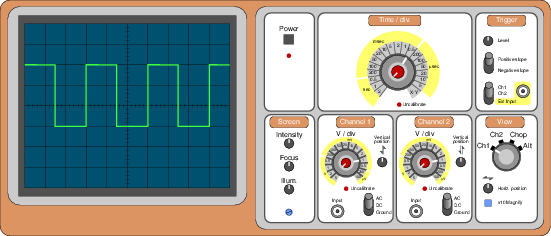Figure 7.2: A simple dual-channel oscilloscope.

For the remainder of this discussion, keep referring back to the drawing of an oscilloscope in Figure 7.2. The first thing to notice is that the display screen is divided into squares – 10 across and 8 down. These squares are called divisions and each is further divided into five sections using little tick marks on the centre lines – the X- and Y-axes. As we’ll see, these divisions are our only reference to the particular characteristics of the waveform.

You basically have control over two parameters on a ‘scope – the speed of the dot as it goes across the screen and the vertical magnification of the voltage. There are a couple of extra controls that we’ll look at one by one. We’ll do this section by section on the panel in Figure 7.2.

##### Power

This section is simple – it contains the Power switch – the on/off button.

##### Screen

Typically, there is a section on the oscilloscope that lets you control some basic aspects of the display like the Intensity of the beam – a measure of its brightness, the focus and the screen Illumination. In addition, there is also probably a small hole showing the top of a screwdriver potentiometer. If this is labeled, it is called the trace rotation. This controls the horizontal angle of the dot on the screen and shouldn’t need to be adjusted very often – once a month maybe.

##### Time / div.

The horizontal speed of the dot is controlled by the knob marked Time/div which stands for “Time per Division.” For example, when this is set to 1 second, it means that the dot will move from left to right, taking 10 seconds to move across the screen (because there are 10 divisions and the dot is traveling at a rate of 1 division per second). As the knob is turned to smaller and smaller divisions of a second, the dot moves faster and faster until it is moving so fast that it appears that there is a line on the screen rather than a single dot.

You’ll notice on the drawing that there are actually two knobs – one gray and one red. The gray one is the usual one. The red one is rarely used so it’s normally in the off position. If it’s turned on, it uncalibrates the larger knob, meaning that if the time-per-division number can no longer be trusted. This is why, when the red knob is turned on, a red light comes on on the front panel next to the word “Uncalibrate,” warning the user that any time readings will be incorrect.

##### Channel 1

Most oscilloscopes that you’ll see have two input channels which can be used to make completely independent measurements of two different signals simultaneously. We’ll just look at one of the channel’s controls, since the second one has identical parameters.

To begin with, the input is a BNC connector, shown on the lower left of the panel. This is normally connected to either of two things. The first is a probe, which has an alligator clip for the ground connection (almost always black) and a pointy part for the probe that you’ll use to make the measurement. The second is somewhat simpler – it’s just two alligator clips, one black one for the ground and one red one for the probe.

The large knob on this panel is marked “V/div” which stands for “Volts per Division.” This is essentially a vertical magnification control, telling you how many divisions the green dot on the screen will move vertically for a given voltage. For example, when this knob is set to 100 mV per division and the dot moves upwards by 2 divisions, then the incoming voltage is 200 mV. A downwards movement of the dot indicates a negative voltage.

This knob can also be uncalibrated using the smaller red knob within it which is normally turned off. Again, there is a warning light to tell you not to trust the display when this knob is turned on.

Moving to the lower right side of the panel, you’ll see a toggle switch that can be used to select three different possibilities – AC, DC and Ground. These don’t mean what your intuition would think, so we’ll go through them. When the toggle is set to Ground, the dot displays the vertical location of 0 V. Since this vertical position is adjustable using the knob just above the toggle switch, it can be set to any location on the screen. Normally, we would set the toggle to Ground which results in a flat horizontal line on the display, then we turn the Vertical position so that the line is perfectly aligned with the centre line on the screen. This means that you have the same positive and negative voltage ranges. If you’re looking at a DC voltage, or an AC voltage with a DC component, then you may want to move the Ground position to a more appropriate vertical location.

When the toggle is set to DC, the dot displays the actual instantaneous voltage. This is true whether the signal is a DC signal or an AC signal. Note that this does not mean that you’re looking at just the DC component of an AC signal. If you have a 1 VRMS sine wave with a -1 V DC offset coming into the oscilloscope and if it’s set to DC mode, then what you see is what you get – both the AC and the DC components of the signal.

When the toggle is set to AC, the dot displays the AC component of the waveform, omitting the DC offset component. So, if you try to measure the voltage of a battery using an oscilloscope in AC mode, then you’ll see a value of 0 V. However, if you’re measuring the ripple of a power supply rail without seeing the DC component, this mode is very useful. For example, let’s say that you’ve got an 18 V rail with a 10 mV of ripple. If the oscilloscope is in DC mode and you set the V/div knob to have a high magnification so that you can accurately measure the 10 mV AC, then you can’t see the signal because it’s somewhere up in the ceiling. If you decrease the magnification so that you can see the signal, it looks like a perfectly flat line because 10 mV AC is so much smaller than 18 V DC. So, you set the mode to AC – this removes the 18 V DC component and lets you look at only the 10 mV AC ripple component at a high magnification.

##### Trigger

Let’s say that you’ve got a 1 kHz sine wave that you’re measuring with the ‘scope. The dot on the screen just travels from left to right at a speed determined by the user. When it gets to the right side of the screen, it just starts on the left side again. Chances are that this rotation doesn’t correspond in any way to the period of the signal. Consequently, the sine wave appears to move on the screen because every time the trace starts, it starts at a new point in the wave. In order to prevent ourselves from getting seasick or at least a headache, we have to tell the oscilloscope to start the trace at the same point in the waveform every time. This is controlled by the trigger. If we set the trigger to a level of 0 V, then the trace will wait until the signal crosses the level of 0 V before moving across the screen. This works great, but we need one more control – the slope. If we just set the trigger level to 0 V and hoped for the best, then sometimes the sine wave would start off on the left side at 0 V heading in the positive direction, sometimes at 0 V in the negative direction. This would result in a shape made of two sine waves, each a vertical mirror image of the other. Therefore, we set the trigger to a particular slope – for example, 0 V heading positive.

We can also select which signal is sent to the triggering circuit. This is done using the toggle switch at the bottom of the panel. The user can select either the signal coming into Channel 1, Channel 2 or a separate External input connected to the BNC connector right on the trigger panel.

##### View

Finally, there is a panel that contains the controls to determine what you’re actually looking at on the screen. There is a knob that permits you to select between looking at either Channel 1, Channel 2 or both simultaneously. There are two options for seeing both signals – Chop and Alternate. There is a slight difference between these two.

In actual fact, there is only one dot tracing across the screen on the ‘scope, so it cannot display both signals simultaneously. It can, however, do a pretty good job of fooling your eyes so that it looks like you’re seeing both signals at once. When the ‘scope is in Alternate mode, the dot traces across the screen showing Channel 1, then it goes back and traces Channel 2, alternating back and forth. If the horizontal speed of the trace is fast enough, then two lines appear on the screen. This works really well for fast traces used for high frequency signals, but if the trace is moving very slowly, then it takes too long to wait for the other channel to re-appear.

Consequently, when you’re using slower trace speeds for lower frequencies, you put the scope in Chop mode. In this case, the dot moves across the screen, hopping back and forth between the Channel 1 and 2 inputs, essentially showing half of each. This doesn’t work well for high trace speeds because the display gets too dim because you’re only showing half of the trace at any given time.

In addition, the View panel also has a couple of other controls. One is a knob which allows you to change the Horizontal position of the traces. In fact, if you turn this knob enough, you should be able to move the trace right off the screen.

The other control is a magnification switch which is rarely used. This is usually a x 10 Magnification which multiplies the vertical magnification of the trace by a factor of 10. Typically, this magnified trace will be shown in addition to the regular trace.

##### A couple of extra things...

There are a couple of things that I’ve left out here. The first is an option on the Time control knob called X-Y mode. This changes the function of the oscilloscope somewhat, turning off the time control of the dot. When this mode is selected, the vertical movement of the dot is determined by the Channel 1 input in a normal fashion. The difference is that the horizontal movement of the dot is determined by the Channel 2 input, positive voltage moving the dot to the right, and negative to the left. This permits you to measure the phase relationship of two signals using what is known as a Lissajous pattern.

The last thing to talk about with oscilloscopes is a minor issue related to grounding. Although the signal input to the ‘scope has an infinite impedance to ground, its ground input is directly connected to the third pin in the AC connector for the device. This means that, if you’re using it to measure typical pro audio gear, the ground on the ‘scope is likely already connected to the ground on the gear through your AC power connections (unless your gear has a floating ground). Consequently, you can’t just put the ground connection anywhere in your circuit as you can with a free-floating DMM.

#### 7.1.4 Function Generators

More often than not, you’ll be trying to measure a piece of audio equipment that doesn’t have an output without a signal being sent to its input. For example, a mixing console or an equalizer doesn’t (or at least shouldn’t) have an output when there’s no input. Consequently, you need something to simulate a signal that would normally be fed into the input portion of the Device Under Test (also known as a DUT). This device is called a Signal Generator or a Function Generator.

A function generator produces an analog voltage output with a waveform, amplitude and frequency determined by the user. In addition, there are also probably controls for adding a DC voltage component to the signal as well as a VCF input for controlling the frequency using an external voltage.

The great thing about a good function generator is that you can set it to a chosen output amplitude and then sweep its frequency without any effect on the output level. You’ll find that this is a very useful characteristic.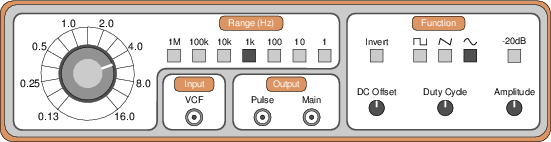Figure 7.3: A simple function generator.

Figure 7.3 shows a simple function generator with a dial-type control. Newer models will have a digital readout which allows the user to precisely set the frequency.

##### Frequency and Range

On the far left of the panel is a dial that is used to control the frequency of the output. This knob usually has a limited range on its own, so it’s used in conjunction with a number of selector switches to determine the range. For example, in this case, if you wanted the output at 12 kHz, then you’d set the dial to 1.2 and press the range button marked 10 k. This would mean 1.2 * 10 k = 12 kHz.

##### Function

On the far right of the panel is a section marked “Function” which contains the controls for various components of the waveform. In the top middle are three buttons for selecting between a square, triangle or sine wave. To the left of this is a button which allows the user to invert the polarity of the signal. On the far right is a button which provides a -20 dB attenuation. This button is used in conjunction with the Amplitude control knob just below it. On the bottom left is the knob which controls the DC Offset. Typically, this is turned off by pushing the knob in and turned on by pulling away from the panel.

The final control here is for the Duty Cycle of the waveform. This is a control which determined the relative time spent in the positive and negative portions of the waveform. For example, a square wave has a duty cycle of 50% because it is high for 50% of the cycle and low for the remaining 50%. If this relationship is changed, the wave approaches a pulse wave. Similarly, a triangle wave has a 50% duty cycle. If this is reduced to 0% then you have a sawtooth wave.

##### Output

The average function generator will have two outputs. One, which is usually marked “Main” is the normal output of the device. The second is a pulse wave with the same frequency as the main output. This can be used for externally triggering an oscilloscope rather than triggering from the signal itself – a technique which is useful when measuring delay times, for example.

##### Input

Finally, some function generators have a VCF input which permits the user to control the frequency of the generator with an external voltage source. This may be useful in doing swept sine tone measurements, where the frequency of the sine wave is swept from a very low to very high frequency to see the entire range of the DUT.

#### 7.1.5 Automated Testing Equipment

Unfortunately, measuring a DUT using these devices is typically a tedious affair. For example, if you wanted to do a frequency response measurement of an equalizer, you’d have to connect a function generator to its input and its output to an oscilloscope. Then you’d have to go through the frequency range of the device, sweeping from a low to a high frequency, checking for changes in the output level on the oscilloscope.

Fortunately, there are a number of various automated testing devices which do all the hard work for you. These are usually computer-controlled systems that use different technqiues to arrive at a reliable measurement of the device. Two of these are the Swept Sine Tone and the Maximum Length Sequence techniques.

NOT YET WRITTEN

#### 7.1.7 MLS-Based Measurements

Back in Section 3.3.2 we looked at a mathematical concept called a maximum length sequence or MLS. An MLS is a series of values (1’s and -1’s) that have a very particular order. We’re not going to look at how these are created – too complicated. We’ll just look at some of their properties. We’ve already seen that they can be used to make diffusion panels for your studio walls. It turns out that they can also be used to make electroacoustic measurements.

Let’s take a small MLS of 15 values (in other words, N = 15). This sequence is shown below.

+ + + - - - + - - + - +

If we were to graph this sequence, it would look like Figure 7.4.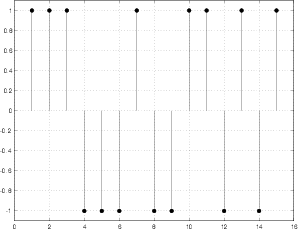Figure 7.4: A graphic representation of the MLS shown above (N=15)

Later, in the section on DSP we’re going to learn about a special mathematical process called a cross-correlation function (This is explained in Section 9.6). For the purposes of this discussion, we’re not going to worry about what that means – as far as we’re concerned for now, it’s math that’s done to a bunch of numbers that result in another bunch of numbers. Basically, a cross-correlation function tells you how much two signals are alike if you compare them to each other at different times.

There’s a wonderful property of an MLS that makes it so special. If you take an MLS and do a cross-correlation function on it with itself, magic occurs. Now, at first it might seem strange to do math to figure out how much a signal is like itself – of course it’s identical to itself... but remember that the cross-correlation function also checks to see how alike the signals are if they are not time-aligned.

Let’s begin by using the MLS with N = 15 shown in Figure 7.4 as a digital audio signal. If we do a cross-correlation function on this with itself, we get the result shown in Figure 7.5.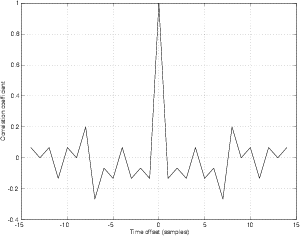Figure 7.5: The cross correlation function of the MLS sequence shown in Figure 7.4 with itself.

What does this tell us? Reading from left to right on the graph, we can see that if the MLS is compared to itself with a time offset of 14 samples, then they have a correlation of about 0.1 which means that they’re not very similar (a correlation of 0 means that they are completely different). As we make the time offset smaller and smaller, the correlation stays very low (less than 0.2) until we get to an offset of 0. At this point, the two signals are identical, so they have a correlation of 1.

If we used a longer MLS sequence, the correlation values at time offsets other than 0 would become very small. For example, Figure 7.6 shows the cross-correlation function of an 8191-point MLS.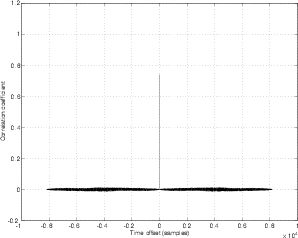Figure 7.6: The cross correlation function of an 8191-point MLS sequence with itself.

If we idealize this graph, we could say that, at a time offset of 0 we have a correlation coefficient of 1, and at all other time offsets, we have a correlation coefficient of 0. If we think of this as a time signal instead of a cross-correlation function, then it’s an impulse. This has some pretty significant implications on our lives.

Let’s say that you wanted to make an impulse response measurement of a room. You’d have to make an impulse, record it and look at the recording. So, you snap your fingers and magically, you can see direct sound, reflections and reverberation in your recording. Unfortunately, this won’t work. You see, in the real world, there’s noise, and if you snap your fingers, you won’t be able to distinguish the finger snap from the noise because your impulse isn’t strong enough – your signal-to-noise ratio is far too small. “No problem!” I hear you cry. “We make a bigger impulse. I’ll get my gun.”

In fact, this would work pretty well. For many years, acousticians carried around pistols and buckets of sand to fire bullets into in order to make impulse response measurements. There are some drawbacks to this procedure, however. You have to own a gun, you need a microphone that can handle a VERY big signal, you need to buy ear protection... All sorts of reasons that work against this option.

There is a better way. Play an MLS out of a loudspeaker. (It will sound a bit like white noise – in fact the signal is sometimes called a pseudo-random signal because it sounds random but it isn’t.) Record the signal in the room with a microphone. Then go home.

When you get home, do a cross-correlation function on the MLS signal that you sent to the loudspeaker with the signal that came into the microphone. When you do, the resulting output will be an impulse response of the room (including the responses of the microphone and the playback system) with a very good signal-to-noise ratio.

Let’s look at a brief example. I made a 1023-point MLS. Then I multiplied by 0.5 delayed it by 500 samples and added the result back to the original. So, we have two overlapping MLS’s with a time offset of 500 samples, and the second one is lowered in level by 6 dB. I then did a cross correlation function of the two signals using the xcorr function in MATLAB. The result is shown in Figure 7.7. As you can see here, the result is a noisy representation of the impulse response of the resulting signal. If I wanted to improve my signal-to-noise ratio, all I have to do is to increase the length of the MLS.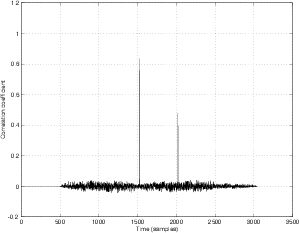Figure 7.7: The cross correlation function of an 1023-point MLS sequence with a modified version of itself (see text for details).

All of this was a lot of work that you don’t have to do because you can buy measurement systems that do all the math for you. The most popular one is the MLSSA system (pronounced “Melissa”) from DRA Laboratories (www.mlssa.com), but other companies make them. For example, the AudioPrecision system has it as an option on their devices (www.ap.com). Of course, if you have MATLAB and the option to playback and record digital audio from your computer, you could just do it yourself.

### 7.2 Electrical Measurements

#### 7.2.1 Output impedance

The output impedance of a device under test (DUT) is measured through the comparison of a given signal being output by the device with and without a load of a known impedance. For example, if we send a 1 kHz sine tone through a DUT and measure its output to be 0 dBV (1 VRMS) with an oscilloscope and no other load, then we put a 600 Ω resistor across the output and measure its new output level (we’ve changed nothing but the load on the output) to be -6.02 dBV (0.5 VRMS), then we know that the DUT has a 600 Ω output impedance at 1 kHz. Effectively, we have built a voltage divider using the output of the DUT and a 600 Ω resistor. The less the output drops when we add the resistor, the lower the output impedance (expect values around 50Ω nowadays...).

The equation for calculating this could be something like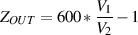(7.1)

Where ZOUT is the output impedance of the DUT (in Ω)

V1 is the level of the unloaded output signal of the DUT (in volts)

and

V2 is the level of the output signal of the DUT loaded with a 600 Ω resistor (in volts)

#### 7.2.2 Input Impedance

The input impedance of a device is measured in a similar fashion to the output impedance, except that we are now using the DUT as the load on a function generator with a known impedance, usually 600 Ω. For example, if we produce a 1 kHz, 0 dBV sine tone with a function generator with a 600 Ω output impedance and the output of the generator drops by 6.02 dB, then we know that the input impedance of the device is 600 Ω.

The equation for calculating this could be something like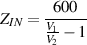(7.2)

Where ZIN is the input impedance of the DUT (in Ω)

V1 is the level of the unloaded output signal of the function generator (with a 600 Ω output impedance) (in volts)

and

V2 is the level of the output signal of the same funtion generator loaded with the input of the DUT (in volts)

#### 7.2.3 Gain

The gain of a DUT is measured by comparing its input to its output. This gain can either be expressed as a multiplier or in decibels.

For example: if the input of a DUT is a 1 kHz sine tone with an amplitude of 100 mV RMS and its output is a 1 kHz sine tone with an amplitude of 1 V RMS, then the gain is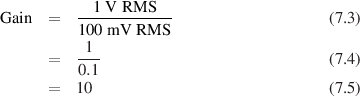This could also be expressed in decibels as follows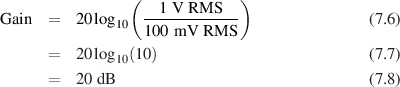#### 7.2.4 Gain Linearity

In theory, a DUT should have the same gain on a signal irrespective of the level of the input (assuming that we’re not measuring a DUT that is supposed to make dynamic gain changes, like a compressor, limiter, expander or gate, for example...). Therefore, the gain, once set to a value of 2 using a 0 dBV 1kHz sine tone should be 2 for all other amplitudes of a 1 kHz sine tone (it may change at other frequencies, as in the case of a filter, for example, but we’ll come to that...) If this is indeed the case, then the DUT is said to have a linear gain response. This will likely not be the case – so we measure the gain of the DUT using various amplitudes at its input. The resulting measurement is most easily graphed on an X-Y plot, with the input amplitude on the x-axis and the deviation from the nominal gain on the y-axis.

This measurement is useful for showing the distortion of low-level signals caused by digital converters in a DUT, for example.

#### 7.2.5 Magnitude Response

A magnitude response measurement is much like a gain linearity measurement with the exception that we are now sweeping through various frequencies, keeping the amplitude of the input signal constant rather than vice versa. This is probably the most common and most commonly abused measurement for audio equipment (for example, when a manufacturer states that a device has a frequency response from 20 Hz to 20 kHz... this means nothing without some indication of a deviation in gain)

We make this measurement by calculating the gain of a DUT at a number of frequencies and plotting them on an X-Y graph, showing frequency (usually plotted logarithmically) on the X-axis and the gain on the Y-axis. The more frequencies we use for the measurement, the more accurate the graph. If we are using a computer based measurement device such as the Audio-Precision or the MLSSA, keep in mind that the frequencies plotted are the result of an FFT-analysis of a time measurement, therefore the frequencies displayed are equally spaced linearly.

Note that most people call this a frequency response measurement, however, this isn’t really correct. A magnitude response only considers the relationship of the amplitude change from input to output. A frequency response measurement actually includes more information like the phase response, described below.

#### 7.2.6 Bandwidth

All DUT’s will exhibit a roll off in their frequency response at low and high frequencies. The bandwidth of the DUT is the width in Hz between the low and high points where we first reach a gain of -3 dB relative to the nominal gain measured at either:

• an arbitrary mid-frequency (usually 1 kHz)
• the highest point in the magnitude response

#### 7.2.7 Phase Response

The phase response of a DUT is similar to the frequency response, with the exception that we are now looking for a change in phase of a signal at various frequencies rather than a change in gain. It is measured by again, comparing the input of a DUT to its output at various frequencies. There are frequently cases when the total delay of the DUT must also be considered in making a phase response measurement as in the case of AD and DA conversion.

Phase response measurements are usually graphed similarly to frequency response measurements, on a semi-log graph, showing frequency on the X-axis and phase on the Y-axis. The phase will usually be plotted in degrees, either wrapped or unwrapped. If the phase is wrapped, then the graph is limited from -180 to 180. Any phase measured to be outside of that window is then wrapped around to the other side of the window – for example, -190 is plotted as 170, since 190 behind and 170 ahead will have the same result. If the plot is unwrapped, the phase will be plotted as measured.

#### 7.2.8 Group Delay

Take a perfect delay unit with a flat frequency response from DC to light, 0% THD, no noise and a delay of 1 ms. If you were to measure the phase response of this device and plot it on a graph that shows phase shift vs. frequency where the latter is plotted on a linear scale, you’d get a straight line. As you get closer and closer to DC, there would be less and less shift, because 1 ms delay is less and less significant. As you get higher in frequency, the phase shift gets greater. This is a device we call a linear phase or phase linear device.

Think about the slope of the phase response plot. It would be a straight line in this case. If we increase the delay time, it would still be a straight line, but with a higher slope. Since the phase response is a straight line, it has no change in slope as we change frequency. This is due to the fact that all frequencies undergo the same amount of delay. Therefore the first derivative (the calculus-heavy way of calculating the slope – see section 1.9) of the plot would be the same number at all frequencies.

However, not all devices behave this way. There are many devices which will delay different frequencies by different amounts of time. The resulting phase response plot of such a device (on a linear frequency scale) would result in a line that is not straight. If that line is not straight, then its slope changes as we change frequency. In other words, the first derivative of the phase response will be frequency dependent. If we were to plot that curve – the slope of the phase response vs. frequency, we would be looking at a plot of the group delay of the device.

Confusing? Maybe... Another way to think of it is that the group delay is the amount by which the phase changes with frequency. If all frequencies have the same delay, then the phase change vs. frequency will be the same at all frequencies (i.e. equal change in degrees of phase shift per Hz). If different frequencies have different delays, then the phase change vs. frequency will be different at different frequencies. An even simpler way to think of group delay intuitively is that it’s a way of thinking about the amount of time it takes a signal with a given frequency to get through your DUT.

#### 7.2.9 Slew Rate

The slew rate of a device, whether it’s a big unit like a mixing console or a little unit like an op amp, is the rate at which it can change from one voltage level to another voltage level. In theory (and in a perfect world), we’d probably like all of our devices to be able to change from any voltage to any other voltage instantly. This, however, is not the case for a number of reasons.

In order to measure the slew rate of a DUT, connect a function generator generating a pulse wave to its input. The greater the amplitude of the signal (assuming that we’re not clipping anything) the easier to make this measurement. Look at the transition between the two voltage levels in the pulse wave both at the input and output of the DUT. Set the scope to a trace time fast enough that the transition no longer looks vertical – this will likely be somewhere in the microsecond range.

If the output of the function generator has instantaneous transitions between voltages, then, looking at the slope of the transition of the output signal, measure the change in voltage in 1 microsecond. This is your slew rate – usually expressed in Volts per microsecond.

#### 7.2.10 Maximum Output

Almost every device has some maximum positive voltage (and minimum negative voltage) level which cannot be exceeded. This level is determined by a number of factors including (but not limited to) the voltage levels of the power supply rails, the operational amplifiers being used, and the gain structure of the device. The principal question in discussing the term “maximum output” is how we define “maximum” – we can say that it’s the absolute maximum voltage which the device is able to output, although this is not usually the case. More often, the maximum output is defined to be the level at which some pre-determined value of distortion of the signal is incurred.

If we send a sine wave into our DUT and start turning things up (either the gain of the DUT or the level of the input signal) while monitoring the output, eventually we’ll reach a level where the DUT is unable to produce a high enough voltage to accurately reproduce the sine waveform (make sure that the fault lies in the DUT and not the function generator). This appears visually as a flattening of the peaks and troughs in the sine wave – called clipping because the wave is “clipped.” If we continue to increase the level, the flat sections will occupy more and more of the period of the signal, approaching a square wave. The more we approach the square wave, the more we are distorting the original shape of the wave. Obviously, the now-flat section of the wave is the maximum level. This is generally not the method of making this measurement.

If we go back to a level just before the wave started clipping and measure the amount of distortion (we’ll talk about how to do this a little later) we’ll find that the distortion of the wave occurs before we can see it on an oscilloscope display. In fact, at the level at which start to see the clipping, you’ve already reached approximately 10% distortion. Usually, the maximum output level is the point when we have either 0.1% or 1% distortion, although this will change from manufacturer to manufacturer. Usually, when a maximum output voltage is given, the percentage of distortion will be included to tell you what the company in question means by the word “maximum.”

Remember that the maximum output of the device is independent of its gain (because we won’t know the level of the input signal). It’s simply a maximum level of the output signal.

#### 7.2.11 Noise measurements

Every device produces noise. Even resistors cause some noise (caused by the molecules moving around due to heat – hence the term thermal noise) If there’s no input (see next paragraph) to a DUT and we measure its output using a true RMS meter, we’ll see a single number expressing its total output noise. This value, however, isn’t all that useful to us, since much of the noise the meter is measuring is outside of the audio range. Consequently, it’s common (and recommended) practice to limit the bandwidth of the noise measurement. Unfortunately, there is no standard bandwidth for this, so you have to rely on a manufacturer to tell you what the bandwidth was. If you’re doing the measurement, pick your frequency band, but tell be sure to include that information when you’re specifying the noise level of the device

How do ensure that there’s no signal coming into a device? This is not as simple as simply disconnecting the previous device. If we just leave the input of our DUT unterminated (a fancy word for “not connected to anything”) then we could be incurring some noise in the device that we normally wouldn’t get if we were plugged into another unit. (Case in point: listen to the hum of a guitar amp when you plug in an input cable that’s not connected to an electric guitar.) Most people will look after this problem by grounding the input – that is to say they just connect the input wire(s) to the ground wire. This is done most frequently, largely due to convenience. If you want to get a more accurate measurement, then you’ll have to terminate the input with an impedance that matches the output impedance of the device that’s normally connected to it.

Remember, when specifying noise measurements, include the bandwidth and the input termination impedance.

#### 7.2.12 Dynamic Range

The dynamic range of a device is the difference in level (usually expressed in dB) between its maximum output level and its noise floor (below which, your audio signal is effectively unusable). This gives you an idea of the absolute limits of the dynamics of the signal you’ll be sending through the DUT. Since a DNR measurement relies in part on the noise floor measurement, you’ll have to specify the bandwidth and input termination impedance.

Also, in spite of everything you see, don’t confuse the dynamic range of a device with its signal to noise ratio.

#### 7.2.13 Signal to Noise Ratio (SNR or S/N Ratio)

The signal to noise ratio is similar to the dynamic range measurement in that it expresses a difference in dB between the noise floor of a DUT and another level. Unlike the dynamic range measurement, however, the signal to noise expresses the difference between the nominal recommended operating level and the noise floor. Usually, this operating level will be shown as 0 dB VU on the meters of the device in question and will correspond at the output to either +4 dBu or -10 dBV, depending on the device.

#### 7.2.14 Equivalent Input Noise (EIN)

There is one special case for noise measurements which occurs when you’re trying to measure the input noise for a microphone preamplifier. In this case, the input noise levels will be so low that they’re difficult to measure accurately (if the input noise levels aren’t too low to measure accurately, then you need a new mic pre...). Luckily, however, we have lots of gain available to us in the preamp itself which we can use to boost the noise in order to measure it. The general idea is that we set the preamp to some known amount of gain, measure the output noise, and then subtract the gain from our noise measurement to calculate what the level is – this is the reason for the “equivalent” in the title, because it’s not actually measured – it’s calculated.

There a couple of things to worry about when making an EIN measurement. The first thing is the gain of the microphone preamplifier. Using a function generator with a very small output level, set the gain of the preamp to +60 dB. This is done by simultaneously measuring the input and the output on an oscilloscope and changing the gain until the output is 1000 times greater in amplitude than the input. Then you disconnect the function generator and the oscilloscope (but don’t change the gain of the preamp!).

Since it’s a noise measurement, we’re concerned with the input impedance. In this case, it’s common practice to terminate the input of the microphone preamp with a 150Ω resistor to match the output impedance of a typical microphone. The easiest way to do this is to solder a 150Ω resistor between pins 2 and 3 of a spare male XLR connector you have lying around the house. Then you can just plug that directly into the mic preamp input with a minimum of hassle.

With the 150 Ω termination connected, and the gain set to +60 dB, measure the level of the noise at the output of the mic preamp using a true RMS meter, with a band-limited input. Take the measurement of the output noise (expressed in dBu) and subtract 60 dB (for the gain of the preamp). That’s the EIN – usually somewhere around -120 dBu.

#### 7.2.15 Common Mode Rejection Ratio (CMRR)

Any piece of “professional” audio gear has what we commonly term “balanced” inputs (be careful – you may not necessarily know what a balanced input is – if you can define it without using the word “impedance” you’ll be wrong). One of the components that makes a balanced input balanced is what is called a differential input. This means that there are two input legs for a single audio signal, one labelled “positive” or “non-inverting” and the other “negative” or “inverting.” If the differential input is working properly, it will subtract the voltage at the inverting input from the voltage at the non-inverting input and send the resulting difference into the DUT. This works well for audio signals because we usually send a balanced input a balanced signal which means (in part) that we’re sending the audio signal on two wires, with the voltages on each of those wires being the negative of the other – so when one wire is at +1 V, the other is at -1 V. The +1 V is sent to the non-inverting input, and the -1 V is sent to the inverting input and the differential input does the math +1 – -1 = 2 and gets the signal out with a gain of 6.02 dB.

The neat thing is that, since any noise picked up on the balanced transmission cable before the balanced input will theoretically be the same on both wires, the differential input subtracts the noise from itself and therefore eliminates it. For more info on this (and the correct definition of “balanced”) see the chapter on grounding and shielding in the electronics section

Since the noise on the cable is common to both inputs (the inverting and the non-inverting) we call it a common mode and since this common mode cancels itself, we say that it has been rejected. Therefore, the ability for a differential input to eliminate a signal sent to both input legs is called the common mode rejection ratio. It’s expressed in dB, and here’s how you measure it.

Take a function generator emitting a sine wave. Send the output of the generator to both input legs of the balanced input of the DUT (pins 2 and 3 on an XLR). Measure the input level and the output level of the DUT. Hopefully, the level of the output will be considerably lower (at least 60 dB lower) than the level of the input. The difference between them, in dB is the CMRR.

This method is the common way to find the CMRR but there is a slight problem with it. Since a balanced input is designed to work best when connected to a balanced output (meaning that the impedances of the two legs to ground are matched – not necessarily that the voltages are symmetrical) the CMRR of our DUT’s input may change if we connected it to unmatched impedances. Two people I have talked to regarding this subject have both commented on the necessity to implement a method of testing the CMRR of a device under unmatched impedances. This may become a recommended standard...

#### 7.2.16 Total Harmonic Distortion (THD)

If you go out to the waveform store and throw out a lot of money on the perfect sine wave, take it home and analyze it, you’ll find that it has very few interesting characteristics beyond its simplicity. The thing that makes it useful to us is that it is an irreducible component – the quarks (NOTE: are quarks irreducible?) of the audio world. What this means is that a sine wave has no energy in any of its harmonics above the fundamental. It is the fundamental and that’s that.

If you then take your perfect sine wave and send it to the input of a DUT and look at the output, you’ll find that the shape of your wave has been modified slightly. Just enough to put some energy in the harmonics above the fundamental. The distortion of the shape of the wave results in energy in the upper harmonics. Therefore, if we measure the total amount of energy in the harmonics relative to the level of the fundamental, we have a quantifiable method of determining how much the signal has been distorted. The greater the amplitude of the harmonics, the greater the distortion.

This measurement is not a common one, but its counterpart, explained below is seen everywhere. It’s made by sending a perfect sine wave into the input of the DUT, and looking at an FFT of the output (which shows the amplitudes of individual frequency “bins”). You add the squares of the amplitudes of the harmonics other than the fundamental, and take the square root of this sum and divide it by the amplitude of the fundamental at the output. If you multiply this value by 100, you get the THD in percent. If you take the log of the number and multiply by 20, you’ll get it in dB.

A couple of things about THD measurements – usually the person doing the measurement will only measure the amplitudes of the harmonics up to a given harmonic, so you’ll see specifications like “THD through to the 7th harmonic” meaning that there is more energy above that, but it’s probably too low or close to the noise floor to measure.

Secondly, unless you have a smart computer which is able to do this measurement, you probably will notice that’s relatively time-consuming and not really worth the effort. This is especially true when you compare the procedure to getting a THD+N measurement as is explained below. That’s why you rarely see it.

#### 7.2.17 Total Harmonic Distortion + Noise (THD+N)

The distortion of a signal isn’t the only reason why a DUT will make things sound worse than when they came in. The noise (meaning wide-band noise as well as hums and buzzes and anything else that you don’t expect) incurred by the signal going through the device is also a contributing factor to signal degradation. As a result, manufacturers use a measurement to indicate the extra energy coming out of the DUT other than the original signal. This is measured using a similar method to the THD measurement, however, it takes considerably less time.

Since we’re looking for all of the energy other than the original, we’ll send a perfect sine wave into the input of the DUT. We then acquire a notch-filter tuned to the frequency of the input sine, which theoretically eliminates the original inputted signal, leaving all other signal (distortion produces and noise which is produced by the DUT itself) to get through. This filtered output is measured using a true RMS meter (we usually band-limit the signal as well, because it’s a noise measurement – see above). This measurement is divided by the RMS level of the fundamental which gives a ratio which can either be converted to a percentage or a dB equivalent as explained above.

#### 7.2.18 Crosstalk

Connect a CD player to an amplifier. In theory, the left channel signal from the CD player goes into the left channel input of the amplifier, gets amplified, and out to the left loudspeaker. In addition, in theory, that’s the only signal that gets to the left loudspeaker. Unfortunately, in reality, that is not the only signal that gets to the speaker. What actually happens is that the amplifier outputs the left channel signal plus a little bit of the right channel signal out the left output. At the same time, a small amount of the left channel signal “bleeds” into the right output.

The amount that the signals bleed into the wrong channel is called a device’s crosstalk because the signals “talk” across to another channel. This happens in all devices for all sorts of reasons. The CD player will have crosstalk at its outputs, the amplifier will have crosstalk between its channels, a mixing console with have crosstalk between input channels and between output channels. Crosstalk exists all the time – the question that you have to ask is “how much signal is bleeding into the wrong channel.”

This is actually a pretty easy thing to measure. Let’s say that you want to measure how much crosstalk there is between the left and right outputs of a mixing console. In other words, if you have a signal that should be only coming out of the left channel, how much is coming out the right?

To measure this, you take a signal (let’s use a 1 kHz sine tone) and make sure that it has a typical level like +4 dBu for a line input (you don’t want it distorting, but you want it to be loud enough to be measurable...). Pan the signal to the left and set the gain of the input and outputs so that you have a throughput gain of 0 dB (therefore the output is +4 dBu). Measure the output levels of the left and right outputs with a true RMS meter. You’ll see that the left output is +4 dBu (because that’s what you made it when you set the gain). The right output should be -∞ dB, but in reality, it will be something low – let’s say something like -90 dBu. That output on the right channel is a combination of the output’s noise and the signal that has bled across from the left channel. The crosstalk measurement is the difference between the two output levels. In this example, that would mean that the crosstalk is -94 dB.

How do we know what portion of the output is the noise and what part is the crosstalk? There are a couple of possibilities...

• If you have already measured the noise floor of the outputs and you know that it’s much lower than the value you’re measuring now, then you know that the biggest contributor to the signal is the crosstalk, and that’s what you’re measuring.
• Another way is to change the input signal level by 6 dB and measure the change in the two outputs. If both go up by 6 dB, then the principal contributor is the crosstalk again. If the crosstalk signal doesn’t increase by as much as 6 dB, then there is contribution from both the noise and the crosstalk. If the crosstalk signal doesn’t change at all, then you’re probably measuring noise and the crosstalk signal is below that.
• The most reliable way is to use a measurement device that can tell you what’s going on at the frequency that you’re measuring, excluding all other information. For example, if you have something that can give you an FFT of the output, then you could use it to measure the crosstalk output at the frequency of the signal you’re inputting. One important thing to remember here is that you will have some component in the device’s noise signal at that frequency as well, so you should do the measurement at multiple signal strengths to see if the output level changes in the same way.

#### 7.2.19 Intermodulation Distortion (IMD)

We have already talked about distortion – this is the characteristic that a device will change (or distort) the shape of a signal and we typically think of it as a bad thing. We measure distortion using a sinusoidal wave as an input in order to make it easy to see the distortion products, however, this is not a very realistic representation of the music we typically hear. The tunes I listen to typically contain more than one frequency at a time.

We have already seen that if you play two sine tones simultaneously, you will hear more than two sine tones. What we haven’t seen yet is the fact that if you play two sine tones simultaneously through a piece of equipment, a type of distortion will occur that results in the equipment outputting more than two sine tones. This is caused by the fact that the two tones can be thought of as causing each other to modulate, and that modulation is distorted, therefore we call the change in signal intermodulation distortion. It’s possible to have a device that has a low distortion characteristic, but has a higher intermodulation distortion value.

CHECK THIS LAST SENTENCE AND DESCRIBE THE STANDARD WAYS TO MEASURE IMD AND WHAT IT SOUNDS LIKE...

#### 7.2.20 DC Offset

Get up in the morning and go to the ocean’s edge. The tide will have some height that is dependent on what time you got there, the phase of the moon, and where you are exactly. Riding on that static water level will be the waves coming in, washing up on the beach. The peaks of the waves will be higher than the “normal” water level. The troughs will be lower.

If you come back to the beach later in the day, the waves might be the same height, but the tide will cause the normal water level to be different.

Now, let’s think of an audio signal as a voltage on a wire. That AC voltage changes over time, but it “rides” on top of a constant level. Usually, that constant level is 0 V, so if the AC wave is symmetrical (meaning that the peak is the same shape and amplitude as the trough), then it will go positive as much as it goes negative.

However, there are some cases in which the AC voltage, like the waves on the ocean, ride on a DC voltage that is not 0 V. One very common example in audio is the output of a microphone that is being phantom-powered. In this case the AC waveform is the microphone’s output. This is sitting on a 48 V DC constant offset that is provided by the microphone preamplifier’s input. In other cases, a DC offset exists where none is desired. This may cause a loss in headroom and therefore dynamic range because one side of the AC waveform may get clipped before the other side. For example, if you have a device that has maximum and minimum voltage rails at ±15 V, and you have a sinusoidal wave that has a 1 V DC offset, then the maximum peak amplitude allowed before the waveform clips at the top will be 14 V. However, this means that the trough of the wave will be 2 V away from clipping at the negative voltage rail.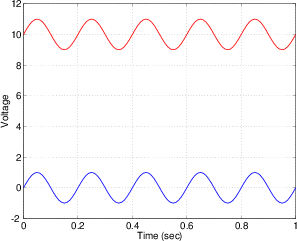Figure 7.8: Two sinusoidal waveforms. Both have a frequency of 5 Hz, and a peak amplitude of 1 V. The blue wave has a 0 V DC offset whereas the red wave “rides” on a 10 V DC offset.

#### 7.2.21 Filter Q

See section 6.1.2.

#### 7.2.22 Impulse Response

NOT YET WRITTEN

DON’T FORGET TO POINT OUT THAT AN IR DOESN’T INCLUDE NON-LINEAR PRODUCTS LIKE DISTORTION

CONVOLUTION

LTI

#### 7.2.23 Cross-correlation

A cross-correlation measurement is, in oversimplified terms, an indication of how similar two signals are. Unfortunately, we’ll need to discuss a couple of other things before we talk about cross-correlation, so it will be discussed in section ??.

#### 7.2.24 Interaural Cross-correlation

As we saw in the previous section, a cross-correlation measurement tells us, frequency band by frequency band, how similar two signals are. If we put a dummy head (a simulated human head with microphones in its ears) in a space and connect the outputs of the microphones in its ears to a machine that measures cross-correlation, then we are making an interaural cross-correlation (or IACC) measurement.

We’ll talk more about the cross-correlation of the outputs of two microphones later, but let’s think a little bit about this now... Put two microphones in a room at about 17 cm apart (the width of your head) and think about how similar their outputs will be. At very low frequencies, the wavelength of the sound in air is much much bigger than the distance between the microphones, therefore the pressure at the two microphones will be almost identical. The lower the frequency, the more similar the two signals and the higher the correlation.

In theory, if the sound source is directly in front of the dummy head, the room is exactly symmetrical, and the head is on the line of symmetry, then the sound at the two ears will be identical. Therefore, the IACC will have a high value at all frequencies. In reality, the signals will never be identical, therefore you will have a varying correlation value at different frequencies.

DOUBLE CHECK ALL THIS TO MAKE SURE I’M ON THE RIGHT TRACK, AND FINISH IT OFF.

### 7.3 Loudspeaker Measurements

Measuring the characteristics of a loudspeaker is not a simple task. There are two basic problems that cause us to be unsure that we are getting a clear picture of what the loudspeaker is actually doing.

The first of these is the microphone that you’re using to make the measurement. The output of the loudspeaker is converted to a measurable electrical signal by a microphone which cannot be a perfect transducer. So, for example, when you make a frequency response measurement, you are not only seeing the frequency response of the loudspeaker, but the response of the microphone as well. The answer to this problem is to spend a lot of money on a microphone that includes extensive documentation of its particular characteristics. In many cases today, this documentation is included in the form of measurement data that can be incorporated into your measurement system and subtracted from your measurements to make the microphone effectively “invisible.”

The second problem is that of the room that you’re using for the measurements. Always remember that a loudspeaker is, more often than not, in a room. That room has two effects. Firstly, different rooms make different loudspeakers behave differently. (Take the extreme case of a large loudspeaker in a small room such as a closet or a car. In this case, the “room” is more like an extra loudspeaker enclosure than a room. This will cause the driver units to behave differently than in a large room because the loudspeaker “sees” the load imposed on the driver by the space itself.) Secondly, the room itself imposes resonance, reflections and reverberation that will be recorded at the microphone position. These effects may be indistinguishable from the loudspeaker (for example, a ringing room mode compared to a ringing loudspeaker driver, or a ringing EQ stage in front of the power amplifier).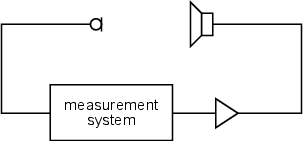Figure 7.9: A simple block diagram showing the basic setup used for typical loudspeaker measurements. The measurement device may be anything from a signal generator and oscilloscope to a sophisticated self-contained automated computer system.

So, our goal in measuring a loudspeaker is to know that we’re actually measuring the loudspeaker itself, and not the characteristics of the measurement microphone or the room in which you’re doing the measurement.

### 7.4 Microphone Measurements

Measuring many characteristics of a microphone cause the same problems as measuring a loudspeaker. Some of the measurements, such as the frequency and phase responses are, of course, highly dependent on the characteristics of the loudspeaker, the room and the placement of the two transducers. However, there are ways around this issue...

In order to do a reliable measurement of a microphone, you’ll need to start with an anechoic chamber to avoid problems caused by reflections. Most anechoic chambers are only reliable at higher frequencies (the lowest trustworthy frequency is dependent on the room) so we can’t trust our measurements in the low end. If you don’t have access to an anechoic chamber, you have three choices. You can either:

• Do your measurements outside on the top of a pole. This is the ultimate anechoic chamber, however it might be a bit noisy.
• Use a measurement system that can ignore surrounding acoustics (such as a MLSSA system).
• Put up with errors in your measurements.

#### 7.4.1 Polar Pattern

As was discussed in detail in Section 6.7, a microphone’s polar pattern is a measurement of its sensitivity (or gain) relative to the angle of incidence of the sound from the sound source. In other words, it tells you what happens to the level of the signal if the microphone is rotated and/or the sound source is moved.

The polar pattern of a microphone shows its sensitivity normalized to the sensitivity of the microphone on-axis. We’ll see a little later how to measure the absolute sensitivity of the microphone, but for a polar pattern measurement we don’t really care what it is.

We start by doing a measurement of the output of the microphone when a sound source is on-axis. This can be done using your preferred method, but the easiest way is probably be using a device that measures the impulse response, that way we can window out early reflections and we can see the frequency-dependent characteristics. Since this is my preferred method, let’s continue assuming that we’re using a MLSSA system.

CONTINUE FROM HERE!

NOT YET WRITTEN